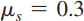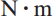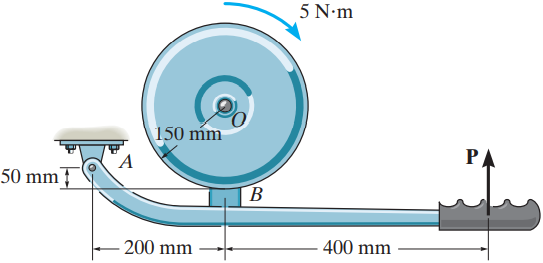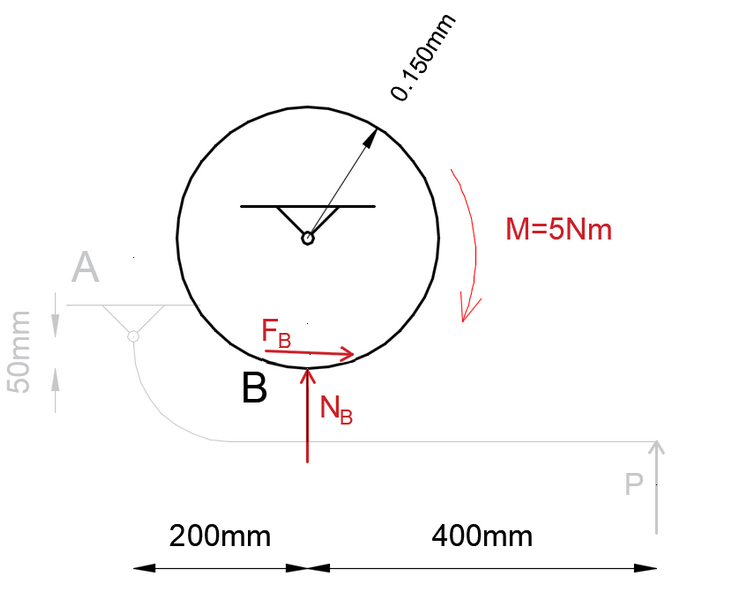×
Get Full Access to Engineering Mechanics: Statics - 14 Edition - Chapter 8 - Problem 8-7
Get Full Access to Engineering Mechanics: Statics - 14 Edition - Chapter 8 - Problem 8-7

×

# The block brake consists of a pin-connected | Ch 8 - 8-7ISBN: 9780133918922 126

## Solution for problem 8-7 Chapter 8

Engineering Mechanics: Statics | 14th Edition

• Textbook Solutions
• 2901 Step-by-step solutions solved by professors and subject experts
• Get 24/7 help from StudySoup virtual teaching assistantsEngineering Mechanics: Statics | 14th Edition

4 5 1 247 Reviews
11
5
Problem 8-7

The block brake consists of a pin-connected lever and friction block at B. The coefficient of static friction between the wheel and the lever is ms = 0.3, and a torque of 5 N # m is applied to the wheel. Determine if the brake can hold the wheel stationary when the force applied to the lever is (a) P = 30 N, (b) P = 70 N. 200 mm 50 mm 400 mm P 150 mm O B A 5 Nm Prob. 87

Step-by-Step Solution:

Problem 8-7

The block brake consists of a pin-connected lever and friction block at B. The coefficient of static friction between the wheel and the lever is, and a torque of 5is applied to the wheel. Determine if the brake can hold the wheel stationary when the force applied to the lever is (a) P = 30 N, (b) P = 70 N.Step by Step Solution

Step 1 of 10

In order for the break to hold the wheel, friction force at B must be less than its maximum friction force(A) First, we’ll isolate and draw the free-body diagram of the wheel .Step 2 of 10

Step 3 of 10

##### ISBN: 9780133918922

Engineering Mechanics: Statics was written by and is associated to the ISBN: 9780133918922. This textbook survival guide was created for the textbook: Engineering Mechanics: Statics, edition: 14. This full solution covers the following key subjects: wheel, lever, Friction, applied, brake. This expansive textbook survival guide covers 11 chapters, and 1136 solutions. Since the solution to 8-7 from 8 chapter was answered, more than 357 students have viewed the full step-by-step answer. The answer to “The block brake consists of a pin-connected lever and friction block at B. The coefficient of static friction between the wheel and the lever is ms = 0.3, and a torque of 5 N # m is applied to the wheel. Determine if the brake can hold the wheel stationary when the force applied to the lever is (a) P = 30 N, (b) P = 70 N. 200 mm 50 mm 400 mm P 150 mm O B A 5 Nm Prob. 87” is broken down into a number of easy to follow steps, and 84 words. The full step-by-step solution to problem: 8-7 from chapter: 8 was answered by , our top Engineering and Tech solution expert on 11/10/17, 05:25PM.

Unlock Textbook Solution